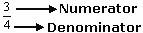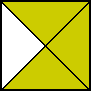Definition Of Numerator

In a fraction, two numbers are separated by a horizontal bar called vinculum. The number written above the vinculum is called Numerator.

In the fraction p/q, the number p is the numerator and the number q is the denominator.
The numerator of the fraction can also be called as the dividend of the fraction.
If the numerator of the fraction is greater than the denominator, then the value of the fraction is greater than 1.
If the numerator of the fraction is less than the denominator, then the value of the fraction is less than 1.

Examples of NumeratorIn the figure given above, the numerator of the unshaded fraction is 1 as the fraction of the unshaded region is 1/4

Solved Example on Numerator

Ques: Identify the numerator of the shaded fraction.A. 5
B. 1
C. 7
D. 6